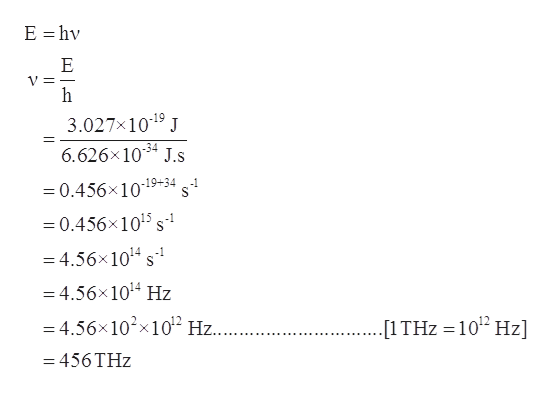# A photon of light produced by a surgical laser has an energy of 3.027 ×× 10−19 J. Calculate the frequency and wavelength of the photon. What is the total energy in 1 mole of photons? What is the color of the emitted light?

Question
42 views

A photon of light produced by a surgical laser has an energy of 3.027 ×× 10−19 J. Calculate the frequency and wavelength of the photon. What is the total energy in 1 mole of photons? What is the color of the emitted light?

check_circle

Step 1

Given information:

Energy of light = 3.027×10-19 J

Step 2

The energy can be expressed as the product of a constant that is plank’s constant(h) and frequency(ν). The mathematical expression for this is given as:

Step 3

Thus, the frequency of light having energy 3.027&time...help_outlineImage TranscriptioncloseE hv Е V = 3.027x1019 J 6.626x1034 J.s -19+34 =0.456x1015 s- =4.56x1014 s =4.56x1014 Hz -4.56x102x1012 Hz.... [1 THz 102 Hz] = 456 THz fullscreen

### Want to see the full answer?

See Solution

#### Want to see this answer and more?

Solutions are written by subject experts who are available 24/7. Questions are typically answered within 1 hour.*

See Solution
*Response times may vary by subject and question.
Tagged in

### Atomic structure